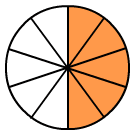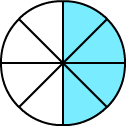# Equivalent Fractions using Models

Rate 0 stars Common Core
Quiz size:
Message preview:
Someone you know has shared Equivalent Fractions using Models quiz with you:

To play this quiz, click on the link below:

https://www.turtlediary.com/quiz/equivalent-fractions-using-models.html

Hope you have a good experience with this site and recommend to your friends too.

Login to rate activities and track progress.
Login to rate activities and track progress.

Equivalent fractions are different fractions that have the same value or represent same part of a whole.

Models represent equivalent fractions if they have the same shape, size, and shaded area.​In the first model, 5 parts out of 10 equal parts are shaded. So, it represents the fraction
5 10
.
In the second model, 4 parts out of 8 equal parts are shaded. So, it represents the fraction
4 8
.

Now, observe that both the models have the same shape and size. Also, the shaded part of the two models are same.​

This means
 5 10
=
 4 8

.

So, the models represent equivalent fractions.

ds

A B C D E F G H I J K L M N O P Q R S T U V W X Y Z